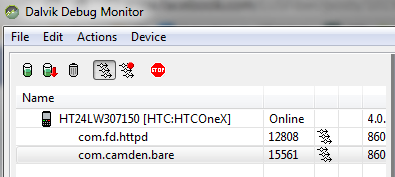This is something I've done many times in the past, but I thought I'd whip up a quick example and share it. Imagine you have a set of data you need to display in columns. With a table it's trivial. You loop and simply begin a new TR every two cells. But what if you aren't using tables? Imagine a CSS based layout with two columns side by side. The contents of the left column need to be every other item, starting with the first one. The contents of the right column need to be every other item, starting with the second one. Here is a quick snippet of code that demonstrates this.

`<cfset data = [1,2,3,4,5,6,7,8,9]>`

`<cfset col1 = []> <cfset col2 = []>`

`<cfloop index="x" from="1" to="#arrayLen(data)#"> <cfset item = data[x]> <cfif x mod 2 is 1> <cfset arrayAppend(col1, item)> <cfelse> <cfset arrayAppend(col2, item)> </cfif> </cfloop>`

I create an initial set of data with 9 items. I then create two arrays - one for each column. Finally I loop over the data set and if odd, add it to column 1, and if even, add it to column two. So to render this I just output my HTML/CSS and loop over each column.

`<style> #left { float: left; width: 200px; } #right { float: left; width: 200px; } </style>`

`<div id="left"> <b>col1</b><br/> <cfloop index="i" array="#col1#"> <cfoutput> #i#<br/> </cfoutput> </cfloop> </div> <div id="right"> <b>col2</b><br/> <cfloop index="i" array="#col2#"> <cfoutput> #i#<br/> </cfoutput> </cfloop> </div>`

Here is how it looks - fancy, eh?